Next: Orbital Angular Momentum Up: Quantum Dynamics Previous: Schrödinger Wave Equation

# Exercises

1. Let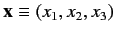be a set of Cartesian position operators, and let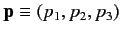be the corresponding momentum operators. Demonstrate that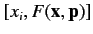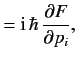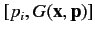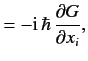where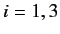, and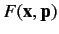,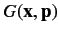are functions that can be expanded as power series.

2. Assuming that the potential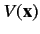is complex, demonstrate that the Schrödinger time-dependent wave equation, (274), can be transformed to give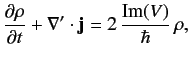where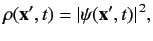and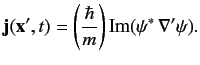3. Consider one-dimensional quantum harmonic oscillator whose Hamiltonian is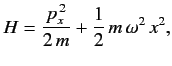whereandare conjugate position and momentum operators, respectively, and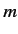,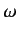are positive constants.

1. Demonstrate that the expectation value of, for a general state, is positive definite.

2. Let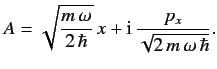Deduce that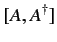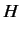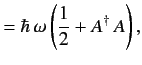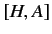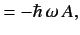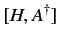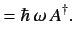3. Suppose that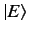is an eigenket of the Hamiltonian whose corresponding energy is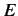: i.e.,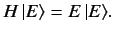Demonstrate that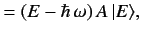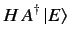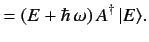Hence, deduce that the allowed values ofare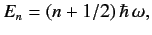where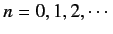.

4. Let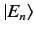be a properly normalized (i.e.,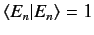) energy eigenket corresponding to the eigenvalue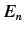. Show that the kets can be defined such that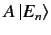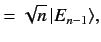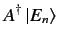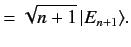Hence, deduce that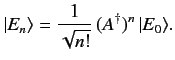5. Let the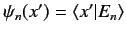be the wavefunctions of the properly normalized energy eigenkets. Given that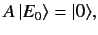deduce that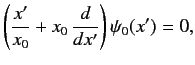where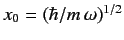. Hence, show that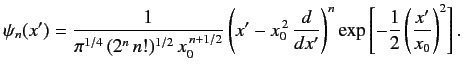4. Consider the one-dimensional quantum harmonic oscillator discussed in Exercise 3. Let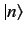be a properly normalized energy eigenket belonging to the eigenvalue. Show that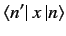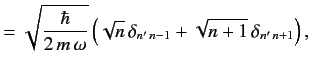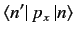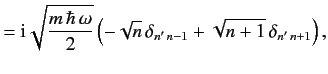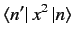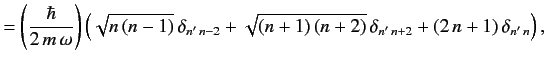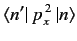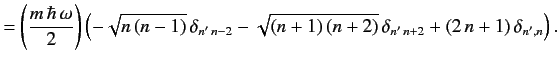Hence, deduce that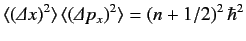for the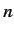th eigenstate.

5. Consider a particle in one dimension whose Hamiltonian is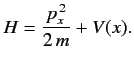By calculating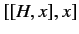, demonstrate that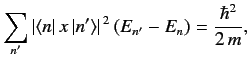whereis a properly normalized energy eigenket corresponding to the eigenvalue, and the sum is over all eigenkets.

6. Consider a particle in one dimension whose Hamiltonian isSuppose that the potential is periodic, such that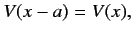for all. Deduce that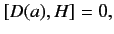where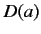is the displacement operator defined in Exercise 4. Hence, show that the wavefunction of an energy eigenstate has the general form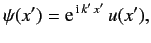where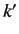is a real parameter, and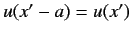for all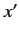. This result is known as the Bloch theorem.

7. Consider the one-dimensional quantum harmonic oscillator discussed in Exercise 3. Show that the Heisenberg equations of motion of the ladder operatorsand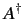are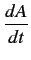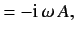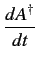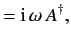respectively. Hence, deduce that the momentum and position operators evolve in time as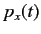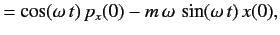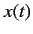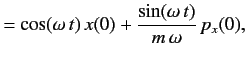respectively, in the Heisenberg picture.

8. Consider a one-dimensional stationary bound state. Using the time-independent Schrödinger equation, prove that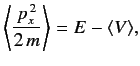and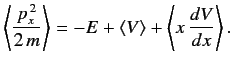[Hint: You can assume, without loss of generality, that the stationary wavefunction is real.] Hence, prove the Virial theorem,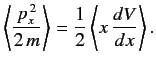Next: Orbital Angular Momentum Up: Quantum Dynamics Previous: Schrödinger Wave Equation
Richard Fitzpatrick 2013-04-08